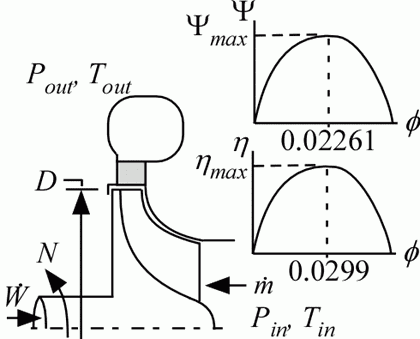Contents - index

Compressor1_CLProcedure Compressor1_CL is a model of a single-stage radial compressor operating outside of the vapor dome.  Pressure rise is determined from a generic head (Y) versus flow coefficient (f) curve that can be shifted vertically and horizontally.  Efficiency is determined from efficiency (h) versus flow coefficient curve that can be shifted vertically and horizontally relative to a generic curve.  The generic curve is based on the radial compressor discussed in Dyreby (2014) and is valid from 0.00544 < f <  0.0512, although surge is likely for f <0.018.  The generic curve provides a maximum efficiency at f = 0.0299 and a maximum head coefficient at f = 0.02261.  The curve can be shifted horizontally using the phi_scale parameter

References:

Dyreby, J., "Modeling the Supercritical Carbon Dioxide Brayton Cycle with Recompression," Ph.D. Thesis, University of Wisconsin, Mechanical Engineering Department, (2014).

Inputs:

T_in:  inlet temperature (K, C, F, or R)

P_in:  inlet pressure (bar, atm, Pa, kPa, MPa)

m_dot:  mass flow rate (kg/s or lb_m/hr)

F\$:  fluid string identifier

N: rotational speed (rev/s)

D:  diameter (ft or m)

eta_max:  maximum isentropic efficiency, typical curve is shifted up to achieve this

psi_max: maximum head coefficient, typical curve is shifted up to achieve this

phi_scale:  scales the eta vs phi and psi vs phi curves horizontally

a value of 1 applies no scaling - peak of efficiency occurs at phi = 0.0299 and peak of psi curve occurs at phi = 0.02261

a value of 1.1 stretches the curve out by 10%

Outputs

P_out:  outlet pressure (bar, atm, Pa, kPa, MPa)

T_out:  outlet temperature (K, C, F, or R)

W_dot:  power (W, kW or Btu/hr)

phi:  flow coefficient

eta:  isentropic efficiency

Ma:  Mach number, evaluated at outlet sound speed

Example:

\$UnitSystem SI Mass J K Pa

T_in=300 [K]

P_in=100000 [Pa]

m_dot=0.1 [kg/s]

F\$='Air'

N=1000 [rev/s]

D=0.1 [m]

eta_max=0.9

psi_max=0.7

phi_scale=1

Call compressor1_cl(T_in, P_in, m_dot, F\$, N, D, eta_max, psi_max, phi_scale: P_out, T_out, W_dot, phi, eta, psi, Ma)

{Solution:

eta=0.8806

Ma=0.8176

phi=0.0349

psi=0.6121

P_out=189623 [Pa]

T_out=368.1 [K]

W_dot=6861 [W]}